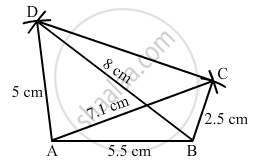Share

# Construct a Quadrilateral Abcd Given Ad = 5 Cm, Ab = 5.5 Cm, Bc = 2.5 Cm, Ac = 7.1 Cm and Bd = 8 Cm. - Mathematics

Course
ConceptConstructing a Quadrilateral When Two Diagonals and Three Sides Are Given

#### Question

Construct a quadrilateral ABCD given AD = 5 cm, AB = 5.5 cm, BC = 2.5 cm, AC = 7.1 cm and BD = 8 cm.

#### Solution

Steps of construction:

Step I: Draw AB = 5 . 5 cm .

Step II: With A as the centre and radius 7 . 1 cm, draw an arc .

Step III : With B as the centre and radius 2 . 5 cm, draw an arc to intersect the arc drawn in Step II at C .

Step IV: With B as the centre and radius 8 cm, draw an arc .

Step V : With A as the centre and radius 5 cm, draw an arc to intersect the arc drawn in Step IV at D .

Step VI: Join DA, DB, BC, AC and CD to obtained the required quadrilateral .Is there an error in this question or solution?

#### APPEARS IN

RD Sharma Solution for Mathematics for Class 8 by R D Sharma (2019-2020 Session) (2017 to Current)
Chapter 18: Practical Geometry (Constructions)
Ex. 18.2 | Q: 5 | Page no. 6
Solution Construct a Quadrilateral Abcd Given Ad = 5 Cm, Ab = 5.5 Cm, Bc = 2.5 Cm, Ac = 7.1 Cm and Bd = 8 Cm. Concept: Constructing a Quadrilateral - When Two Diagonals and Three Sides Are Given.
S